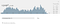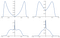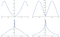Probability distributions are incredible useful tools, but are hard to grapple with intuitively. Often, seemingly benign questions can have very surprising results. With Guesstimate, these questions can be rapidly explored, exposing unexpected facets of statistics mathematically interesting in their own right.

One such question is the following:

What happens if you take a normal random variable, and shrink it down into the interval (-1,1)? Here, by “shrink it down” I mean condense the entire real line, and with it the range of the random variable, into the interval (-1,1) in a monotonic fashion, so zero stays at zero, and ±∞ ↦ ±1. What does the PDF of this strange new distribution look like?

Let’s make this a bit more specific. We’ll take the standard normal random variable, Z, and operate on it via

(note that this has the property that 0 ↦ 0, ±∞ ↦±1, as desired).

Who thinks the resulting PDF is:

1. Peaked at zero, decaying towards ±1 in a “condensed Bell curve”?
2. Lightly peaked at zero, decaying towards ±1, then sharply rising towards infinity near ±1?
3. Bimodal, with no peak at zero but two symmetric peaks reflected about zero, before decaying towards ±1?

The answer to this poll, which I found quite surprising, is a definitive 3! Playing with this in Guesstimate, (with CG being a standard normal) we see:A shrunk standard normal shows bimodal behavior

The full shape of the true PDF isn’t totally clear with only 5000 samples, but this still gives us a general idea of the bimodality of the resulting distribution.

# Getting Abnormal

We can push a few other distributions through this process, and use a few more functions:

Let’s note two things here:

First, distributions with no defined (think infinite) expected value (the Cauchy and Student’s T parametrized at 1) have notably more weight towards the edges of the interval (-1, 1) when shrunk than do distributions with well defined expected values. This makes sense, as distributions that get ‘closer to infinity’ should get ‘closer to ±1’ when shrunk.

Second, When we shrink using functions of the form

we see no central peak for p<1 and a definite central peak for p>1. Perhaps there is some critical value here.

Samples are really great for quickly talking about this behavior, but what are the real PDFs here? In general, they aren’t easy to compute analytically, but we can extract them in some cases. Consider the below, extracted using Mathematica:PDFs for the ‘Shrunk Normal’. From top left to bottom right, shrunk by: sgn(x)(|x|^0.5)/(|x|^0.5+1), x/(|x|+1), sgn(x)(|x|^(1.5))/(|x|^(1.5)+1), sgn(x) x^2/(x^2+1)

Here, we see the bimodal behavior is definitely an existent phenomena; not an emergent property from sampling error. Additionally, we can see that in the top row, where p1 shows no peak near zero, but when p>1, there is a notable nub at zero, again agreeing with our sampled histograms.

What about for our pathological friend the Cauchy distribution?PDFs for the ‘Shrunk Cauchy’. From top left to bottom right, shrunk by: sgn(x)(|x|^0.5)/(|x|^0.5+1), x/(|x|+1), sgn(x)(|x|^(1.5))/(|x|^(1.5)+1), sgn(x) x^2/(x^2+1)

Here, it is interesting to note how much more weight is, on average, near ±1 than in the case of the Shrunk Normal. Again, we seem the same critical value behavior, but the extremes are more pronounced in each case. Additionally, here, we start to see some divergence from our guesstimate drawn results; notably, the peaks we see near ±1 in our sampled case don’t appear in these real PDFs. There are two possible reasons for this:

1. Sampling from pathological distributions is actually rather difficult to do robustly, and our strategy could be over representing large samples.
2. Mathematica’s analytical solution to the PDF may be inaccurate (as several of these did not converge within typical boundaries).

# Key Insights

Shrinking distributions is neither as simple nor direct as it seems. Walking into this investigation, I expected the shrunk normal distribution to appear simply like a shrunk Bell Curve, but the real result is much more complicated (hooray for the chain rule). Moreover, it seems we can use this method to gain some semblance of a qualitative feel for ‘how big’ a distribution gets; Cauchy and Student’s T distributions both reach much closer to ±1 than does the normal distribution.

There’s much more to be learned through intuitive exploration of statistical distributions; to check out the examples here in more depth, and see some stuff we left out, go to the underlying model and play with it yourself!

Written by

## The Guesstimate Blog

#### A spreadsheet for things that aren’t certain

Welcome to a place where words matter. On Medium, smart voices and original ideas take center stage - with no ads in sight. Watch
Follow all the topics you care about, and we’ll deliver the best stories for you to your homepage and inbox. Explore
Get unlimited access to the best stories on Medium — and support writers while you’re at it. Just \$5/month. Upgrade